# Samacheer Kalvi 12th Chemistry Solutions Chapter 4 Transition and Inner Transition Elements

Tamilnadu State Board Solutions for Class 12th Chemistry Solutions Chapter 4 Transition and Inner Transition Elements Questions and Answers will help you to improve the complete subject knowledge. Every student must look at the single concept included in Samacheer Kalvi 12th Chemistry Solutions Chapter 4 Transition and Inner Transition Elements Book Solutions Answers Guide Solutions PDF. All the concepts are explained clearly with examples and pictures.

Students can easily avoid the struggle to get the best book for Chemistry Solutions Chapter 4 Transition and Inner Transition Elements Book Solutions Answers Guide learning. You have to go through Chapterwise Samacheer Kalvi Class 12th Textbook Solutions for Chemistry Solutions Chapter 4 Transition and Inner Transition Elements Book Solutions for better practice.

## Tamilnadu Samacheer Kalvi 12th Chemistry Solutions Chapter 4 Transition and Inner Transition Elements

Students who wish to have the strong basics of Chemistry Solutions Chapter 4 Transition and Inner Transition Elements can use Tamilnadu State Board Class 12th Chemistry Solutions Chapter 4 Transition and Inner Transition Elements Questions and Answers Guide pdf. Enhance your knowledge by referring to the Samacheer Kalvi Solutions pdf. We provided a free pdf of Tamilnadu State Board Class 12th Chemistry Solutions Chapter 4 Transition and Inner Transition Elements Book Solutions Answers Guide material and textbook for students. Check it out now and start preparing for the exam immediately. Score maximum marks in the exam by referring to Samacheer Kalvi Class 12th Chemistry Solutions Chapter 4 Transition and Inner Transition Elements Book Solutions Answers Guide Solutions Pdf.

### Samacheer Kalvi 12th Chemistry Transition and Inner Transition Elements Text Book Evalution

12th Chemistry Chapter 4 Book Back Answers Questions 1.
Sc ( Z=21) is a transition element but Zinc (z=30) is not because ……………..
(a) both Sc3+ and Zn2+ ions are colourless and form white compounds.
(b) in case of Sc, 3d orbital are partially filled but in Zn these are completely filled
(c) last electron as assumed to be added to 4s level in case of zinc
(d) both Sc and Zn do not exhibit variable oxidation states
(c) in case of Sc, 3d orbital are partially filled but in Zn these are completely filled

12th Chemistry Unit 4 Book Back Answers Question 2.
Which of the following d block element has half filled penultimate d sub shell as well as half filled valence sub shell?
(a) Cr
(b) Pd
(c) Pt
(d) none of these
(a) Cr
Hint: Cr ⇒ [Ar]3d5 4s1

Transition And Inner Transition Elements Class 12 Question 3.
Among the transition metals of 3d series, the one that has highest negative (M2+/ M) standard electrode potential is ……………..
(a) Ti
(b) Cu
(c) Mn
(d) Zn
(a) Ti

Transition And Inner Transition Elements Pdf Question 4.
Which one of the following ions has the same number of unpaired electrons as present in V3+?
(a) Ti3+
(b) Fe3+
(c) Ni2+
(d) Cr3+
(c) Ni2+

12th Chemistry Chapter 4 Question 5.
The magnetic moment of Mn2+ ion is ……………..
(a) 5.92BM
(b) 2.80BM
(c) 8.95BM
(d) 3.90BM
(a) 5.92BM
Hint: Mn2+ ⇒ 3d contains 5 unpaired electrons
n = 5
= $$\sqrt { n(n+2) } BM$$
= $$\sqrt { 5(5+2) }$$ = $$\sqrt { 35 }$$ = 5.92 BM

Transition Elements Notes Pdf Question 6.
Which of the following compounds is colourless?
(a) Fe3+
(6) Ti4+
(c) CO2+
(d) Ni2
(b) Ti4+
Hint: Ti4+ contains no unpaired electrons in d orbital, hence no d-d transition.

Samacheer Kalvi Guru 12th Chemistry Question 7.
The catalytic behaviour of transition metals and their compounds is ascribed mainly due to ……………..
(a) their magnetic behaviour
(b) their unfilled d orbitals
(c) their ability to adopt variable oxidation states
(d) their chemical reactivity
(c) their ability to adopt variable oxidation states

12th Chemistry Samacheer Kalvi Question 8.
The correct order of increasing oxidizing power in the series ……………..
(a) $${ VO }_{ 2 }^{ + }$$ < $${ Cr }_{ 2 }{ O }_{ 7 }^{ 2- }$$ < $$Mn{ O }_{ 4 }^{ – }$$
(b) $${ Cr }_{ 2 }{ O }_{ 7 }^{ 2- }$$ < $${ VO }_{ 2 }^{ + }$$ < $$Mn{ O }_{ 4 }^{ – }$$
(c) $${ Cr }_{ 2 }{ O }_{ 7 }^{ 2- }$$ < $$Mn{ O }_{ 4 }^{ – }$$ < $${ VO }_{ 2 }^{ + }$$
(d) $$Mn{ O }_{ 4 }^{ – }$$ < $${ Cr }_{ 2 }{ O }_{ 7 }^{ 2- }$$ < $${ VO }_{ 2 }^{ + }$$
(a) $${ VO }_{ 2 }^{ + }$$ < $${ Cr }_{ 2 }{ O }_{ 7 }^{ 2- }$$ < $$Mn{ O }_{ 4 }^{ – }$$
Hint: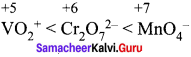greater the oxidation state, higher is the oxidising power.

Samacheer Kalvi 12th Chemistry Question 9.
The alloy of copper that contain Zinc is ……………..
(a) Monel metal
(b) Bronze
(c) bell metal
(d) brass
(d) brass

Samacheer Kalvi 12th Chemistry Solutions Question 10.
Which of the following does not give oxygen on heating?
(a) K2Cr2O7
(b) (NH4)2Cr2O7
(c) KClO3
(d) Zn(ClO3)2
(b) (NH4)2Cr2O7
Hint: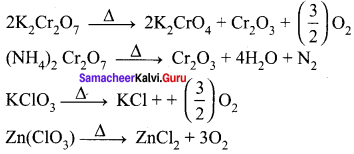Samacheerkalvi.Guru 12th Chemistry Question 11.
In acid medium, potassium permanganate oxidizes oxalic acid to ……………..
(a) Oxalate
(b) Carbon dioxide
(c) acetate
(d) acetic acid
(b) Carbon dioxide
Hint: $${ 5(COO) }_{ 2 }^{ 2- }$$ + $${ 2MnO }_{ 4 }^{ – }$$ + 16H+ $$\underrightarrow { \triangle }$$ 2Mn2+ + 10CO2+$$\uparrow$$ + 8H2O

12 Chemistry Samacheer Kalvi Question 12.
Which of the following statements is not true?
(a) on passing H2S, through acidified K2Cr2O7 solution, a milky colour is observed.
(b) Na2Cr2O7 is preferred over K2Cr2O7 in volumetric analysis
(c) K2Cr2O7 solution in acidic medium is orange in colour
(d) K2Cr2O7 solution becomes yellow on increasing the pH beyond 7
(b) Na2Cr2O7 is preferred over K2Cr2O7 in volumetric analysis

Transition And Inner Transition Elements Class 12 Exercise Question 13.
Permanganate ion changes to in acidic medium ……………..
(a) $${ MnO }_{ 4 }^{ 2- }$$
(b) Mn2+
(c) Mn3+
(d) MnO2
(b) Mn2+
Hint: $${ MnO }_{ 4 }^{ – }$$ + 8H+ + 5e → Mn2+ + 4H2O

Inner Transition Elements Pdf Question 14.
A white crystalline salt (A) react with dilute HCl to liberate a suffocating gas (B) and also forms a yellow precipitate . The gas (B) turns potassium dichromate acidified with dil H2SO4 to a green coloured solution(C). A,B and C are respectively ……………..
(a) Na2SO3, SO2, Cr2(SO4)3
(b) Na2S2O3, SO2, Cr2(SO4)3
(c) Na2S, SO2, Cr2(SO4)3
(d) Na2SO4, SO2, Cr2(SO4)3
(a) Na2SO3, SO2, Cr2(SO4)3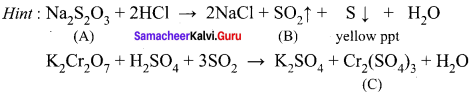Question 15.
$${ MnO }_{ 4 }^{ – }$$ react with Br in alkaline PH to give ……………..
(a) $${ BrO }_{ 3 }^{ – }$$, MnO2
(b) Br3, $${ MnO }_{ 4 }^{ 2- }$$
(c) Br3, MnO3
(d) BrO, $${ MnO }_{ 4 }^{ 2- }$$
(a) $${ BrO }_{ 3 }^{ – }$$, MnO2
Hint: $${ 2MnO }_{ 4 }^{ – }$$ + Br- + H2O → 2OH- + 2MnO2 + $${ BrO }_{ 3 }^{ – }$$

Question 16.
How many moles of I2 are liberated when 1 mole of potassium dichromate react with potassium iodide?
(a) 1
(b) 2
(c) 3
(d) 4
(c) 3
Hint: K2Cr2O7 + 6KI + 7H2SO4 → 4K2SO4 + Cr2 (SO4)3 + 7H2O + 3I2

Question 17.
The number of moles of acidified KMnO4 required to oxidize 1 mole of ferrous oxalate(FeC2O4) is …………..
(a) 5
(b) 3
(c) 0.6
(d) 1.5
(c) 0.6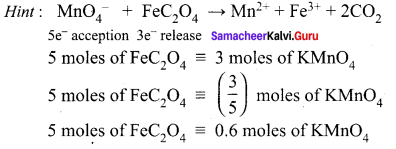Question 18.
When a brown compound of Mn (A) ids treated with HCl, it gives a gas (B). The gas (B) taken in excess reacts with NH3 to give an explosive compound (C). The compound A, B and C are ……………
(a) MnO2, Cl2, NCl3
(b) MnO, Cl2, NH4Cl
(c) Mn3O4, Cl2, NCl3
(d) MnO3, Cl2, NCl2
(a) MnO2, Cl2, NCl3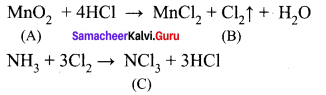Question 19.
Which one of the following statements related to lanthanons is incorrect?
(a) Europium shows +2 oxidation state.
(b) The basicity decreases as the ionic radius decreases from Pr to Lu.
(c) All the lanthanons are much more reactive than aluminium.
(d) Ce4+ solutions are widely used as oxidising agents in volumetric analysis.
(c) All the lanthanons are much more reactive than aluminium.
Hint: As we move from La to Lu , their metallic behaviour because almost similar to that of aluminium.

Question 20.
Which of the following lanthanoid ions is diamagnetic?
(a) Eu2+
(b) Yb2+
(c) Ce2+
(d) Sm2+
(6) Yb2+
Hint: Yb2+ – 4f14 – no unpaired electrons – diamagnetic

Question 21.
Which of the following oxidation states is most common among the lanthanoids?
(a) 4
(b) 2
(c) 5
(d) 3
(d) 3

Question 22.
Assertion: Ce4+ is used as an oxidizing agent in volumetric analysis.
Reason: Ce4+ has the tendency of attaining +3 oxidation state.
(a) Both assertion and reason are true and reason is the correct explanation of assertion.
(b) Both assertion and reason are true but reason is not the correct explanation of assertion.
(c) Assertion is true but reason is false. .
(d) Both assertion and reason are false.
(a) Both assertion and reason are true and reason is the correct explanation of assertion.

Question 23.
The most common oxidation state of actinoids is ……………
(a) +2
(b) +3
(c) +4
(d) +6
(c) +4

Question 24.
The actinoid elements which show the highest oxidation state of +7 are ……………
(a) Np, Pu, Am
(b) U, Fm, Th
(c) U, Th, Md
(d) Es, No, Lr
(a) Np, Pu, Am

Question 25.
Which one of the following is not correct?
(a) La(OH)2 is less basic than Lu(OH)3
(b) In lanthanoid series ionic radius of Ln3+ ions decreases
(c) La is actually an element of transition metal series rather than lanthanide series
(d) Atomic radii of Zr and Hf are same because of lanthanide contraction
(a) La(OH)2 is less basic than Lu(OH)3

Question 1.
What are transition metals? Give four examples.

1. Transition metal is an element whose atom has an incomplete d sub shell or which can give rise to cations with an incomplete d sub shell.
2. They occupy the central position of the periodic table, between s-block and p-block elements.
3. Their properties are transitional between highly reactive metals of s-block and elements of p-block which are mostly non metals.
4. Example – Iron, Copper, Tungsten, Titanium.

Question 2.
Explain the oxidation states of 3d series elements.
1. The first transition metal Scandium exhibits only +3 oxidation state, but all other transition elements exhibit variable oxidation states by losing electrons from (n-l)d orbital and ns orbital as the energy difference between them is very small.

2. At the beginning of the 3d series, +3 oxidation state is stable but towards the end +2 oxidation state becomes stable.

3. The number of oxidation states increases with the number of electrons available, and it decreases as the number of paired electrons increases. For example, in 3d series, first element Sc has only one oxidation state +3; the middle element Mn has six different oxidation states from +2 to +7. The last element Cu shows +1 and +2 oxidation states only.

4. Mn2+ (3d5) is more stable than Mn4+ (3d3) is due to half filled stable configuration.

Question 3.
What are inner transition elements?
1. The inner transition elements have two series of elements.

• Lanthanoids
• Actinoids

2. Lanthanoid series consists of 14 elements from Cerium (58Ce) to Lutetium (71Lu) following Lanthanum (57La). These elements are characterised by the preferential filling of 4f orbitals. .

3. Actinoids consists of 14 elements from Thorium (90Th) to Lawrencium (103Lr) following Actinium (89Ac). These elements are characterised by the preferential filling of 5f orbital.

Question 4.
Justify the position of lanthanides and actinides in the periodic table.
1. In sixth period after lanthanum, the electrons are preferentially filled in inner 4f sub shell and these 14 elements following lanthanum show similar chemical properties. Therefore these elements are grouped together and placed at the bottom of the periodic table. This position can be justified as follows.

• Lanthanoids have general electronic configuration [Xe] 4f2-14 5d0-1 6s2
• The common oxidation state of lanthanoids is +3
• All these elements have similar physical and chemical properties.

2. Similarly the fourteen elements following actinium resemble in their physical and chemical properties.

3. If we place these elements after Lanthanum in the periodic table below 4d series and actinides below 5d series, the properties of the elements belongs to a group would be different and it would affect the proper structure of the periodic table.

4. Hence a separate position is provided to the inner transition elements at the bottom of the periodic table.

Question 5.
What are actinoides? Give three examples.

1. The fourteen elements following actinium ,i.e., from thorium (Th) to lawrentium (Lr) are called actinoids.
2. All the actinoids are radioactive and most of them have short half lives.
3. The heavier members being extremely unstable and not of natural occurrence. They are produced synthetically by the artificial transformation of naturally occuring elements by nuclear reactions.
4. Example – Thorium, Uranium, Plutonium, Californium.

Question 6.
Why Gd3+ is colourless?
Gd – Electronic Configuration: [Xe] 4f7 5d1 6s2
Gd3+ – Electronic Configuration: [Xe] 4f7
In Gd3+, no electrons are there in outer d-orbitals. d-d transition is not possible. So it is colourless.

Question 7.
Explain why compounds of Cu2+ are coloured but those of Zn2+ are colourless.
Cu (Z = 29) Electronic configuration is [Ar] 3d10 4s1
Cu2+: Electronic configuration is [Ar] 3d9.
In Cu2+, promotion of electrons take place in outer d-orbital by the absorption of light form visible region involves d-d transition. Due to this Cu2+ compounds are coloured. Where in Zn2+ electronic configuration is [Ar]3d10. It has completely filled d-orbital. So there is no chance of d – d transition. So Zn2+ compounds are colourless.

Question 8.
Describe the preparation of potassium dichromate.
Preparation of potassium dichromate:
1. Potassium dichromate is prepared from chromite ore. The ore FeO. Cr2O3 is concentrated by gravity separation process.

2. The concentrated ore is mixed with excess sodium carbonate and lime and roasted in a reverbratory furnace.
4FeCr2O4 + 8Na2CO3 + 7O2 $$\underrightarrow { 900-{ 1000 }^{ 0 }C }$$ 8 Na2CrO4 + 2Fe2O3 + 8CO2$$\uparrow$$

3. The roasted mass is treated with water to separate soluble sodium chromate from insoluble iron oxide. The yellow solution of sodium chromate is treated with concentrated sulphuric acid which converts sodium chromate into sodium dichromate.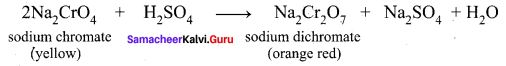4. The above solution is concentrated to remove less soluble sodium sulphate. The resulting solution is filtered and concentrated. It is cooled to get the crystals of Na2SO2.2H2O.

5. The saturated solution of sodium dichromate in water is mixed with KCl and then concentrated to get crystals of NaCl. It is filtered while hot and the filtrate is cooled to obtain K2Cr2O7 crystals.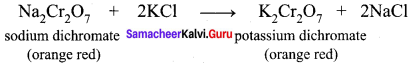Question 9.
What is lanthanide contraction and what are the effects of lanthanide contraction?
As we move across 4f series, the atomic and ionic radii of lanthanoids show gradual decrease with increase in atomic number. This decrease in ionic size is called lanthanoid contraction.
Effects (or) Consequences of lanthanoid contraction:
1. Basicity differences: As we move from Ce3+ to Lu3+ , the basic character of Ln3+ ions decrease. Due to the decrease in the size of Ln3+ ions, the ionic character of Ln – OH bond decreases (covalent character increases) which results in the decrease in the basicity.

2. Similarities among lanthanoids – In the complete f-series only 10 pm decrease in atomic radii and 20 pm decrease in ionic radii is observed. Because of this very small change in radii of lanthanoids, their chemical properties are quite similar.

The elements of second and third transition series resemble each other more closely than the elements of first and second transition series due to lanthanoid contraction. For example,

• 4d series – Zr – Atomic radius 145 pm
• 5d series – Hf – Atomic radius 144 pm

Question 10.
Complete the following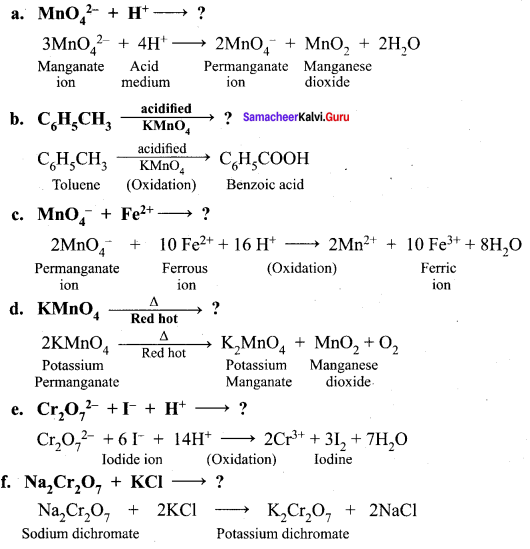Question 11.
What are interstitial compounds?

1. An interstitial compound or alloy is a compound that is formed when small atoms like hydrogen, boron, carbon or nitrogen are trapped in the interstitial holes in a metal lattice.
2. They are usually non-stoichiometric compounds.
3. Transition metals form a number of interstitial compounds such as TiC, ZrH1.92, Mn4N etc.
4. The elements that occupy the metal lattice provide them new properties.
• They are hard and show electrical and thermal conductivity
• They have high melting points higher than those of pure metals
• Transition metal hydrides are used as powerful reducing agents
• Metallic carbides are chemically inert.

Question 12.
Calculate the number of unpaired electrons in Ti3+, Mn2+ and calculate the spin only magnetic moment.
Ti3+:
Ti (Z = 22). Electronic configuration [Ar] 3d2 4s2
Ti3+ – Electronic configuration [Ar] 3d1
So, the number of unpaired electrons in Ti3+ is equal to 1.
Spin only magnetic moment of Ti3+ = $$\sqrt { 1(1+2) }$$ = $$\sqrt { 3 }$$ = 1.73µB

Mn2+:
Mn (Z = 25). Electronic configuration [Ar] 3d5 4s2
Mn2+ – Electronic configuration [Ar] 3d5
Mn2+ has 5 unpaired electrons.
Spin only magnetic moment of Mn2+ = $$\sqrt { 5(5+2) }$$ = $$\sqrt { 35 }$$ = 5.91µB

Question 13.
Write the electronic configuration of Ce4+ and CO2+.
Ce (Z = 58) → Ce4+4e
Ce4+ – Is2 – 2s22p63s23p64s23d104p6 5s24d10 5p6
CO2+ – Is22s22p63s23p64s23d5.

Question 14.
Explain briefly how +2 states becomes more and more stable in the first half of the first row transition elements with increasing atomic number.
First transition series.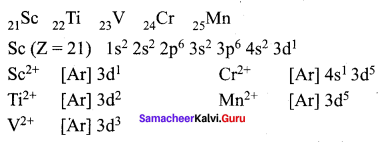Question 15.
Which is more stable? Fe3+ or Fe2+ – explain.
Fe (Z = 26)
Fe → Fe2+ + 2e
Fe → Fe3+ + 3e
Fe2+ [Number of electrons 24]
Electronic configuration = [Ar]3d6
Fe3+ [Number of electrons 23]
Electronic configuration = [Ar]3d5
Among Fe3+ and Fe2+, Fe3+ is more stable due to half filled d-orbital. This can be explained by Aufbau principle. Half filled and completely filled d-orbitals are more stable than partially filled d-orbitals. So Fe3+ is more stable than Fe2+.

Question 16.
Explain the variation in E0M2+/M3+3d series.
1. In transition series, as we move down from Ti to Zn, the standard reduction potential E0M2+/M3 value is approaching towards less negative value and copper has a positive reduction potential, i.e. elemental copper is more stable than Cu2+.

2. E0M2+/M value for manganese and zinc are more negative than regular trend. It is due to extra stability arises due to the half filled d5 configuration in Mn2+ and completely filled d10 configuration in Zn2+.

3. The standard electrode potential for the M3+ / M2+ half cell gives the relative stability between M3+ and M2+.

4. The high reduction potential of Mn3+ / Mn2+ indicates Mn2+ is more stable than Mn3+.

5. For Fe3+ / Fe2+ the reduction potential is 0.77 V, and this low value indicates that both Fe3+ and Fe2+ can exist under normal condition.

6. Mn3+ has a 3d2 configuration while that of Mn2+ is 3d5. The extra stability associated with a half filled d sub-shell makes the reduction of Mn3+ very feasible [E° = +1.51 V]

Question 17.
Compare lanthanides and actinides.
Lanthanoids:

1. Differentiating electron enters in 4f orbital
2. Binding energy of 4f orbitals are higher
3. They show less tendency to form complexes
4. Most of the lanthanoids are colourless
5. They do not form oxo cations
6. Besides +3 oxidation states lanthanoids show +2 and +4 oxidation states in few cases.

Actinoids:

1. Differentiating electron enters in 5f orbital
2. Binding energy of 5f orbitals are lower
3. They show greater tendency to form complexes
4. ost of the actinoids are coloured.
E.g : U3+ (red), U4+ (green), $${ UO }_{ 2 }^{ 2+ }$$(yellow)
5. They do form oxo cations such as $${ UO }_{ 2 }^{ 2+ }$$ $${ NpO }_{ 2 }^{ 2+ }$$ etc.
6. Besides +3 oxidation states actinoids show higher oxidation states such as +4, +5, +6 and +7.

Question 18.
Explain why Cr2+ is strongly reducing while Mn3+ is strongly oxidizing.
Cr2+ is strong reducing while Mn3+ is strongly oxidising.
E0Cr3+/Cr2+ is -0.41 V
Cr2+ + 2e → Cr E° = – 0.91V.
If the standard electrode potential E° of a metal is large and negative, the metal is a powerful reducing agent because it loses electrons easily.
Mn → Mn3+ + 3e
Mn3+ + e → Mn2+

Mn3+ [Ar]3d4
E° = + 1.51 V
If the standard electrode potential E° of a metal is large and positive, the metal is a powerful oxidising agent because it gains electrons easily.

Question 19.
Compare the ionization enthalpies of first series of the transition elements. Ionization enthalpies of first transition series:

1. Ionization energy of transition element is intermediate between those of s and p block elements.
2. As we move from left to right in a transition metal series, the ionization enthalpy increases as expected. This is due to increase in nuclear charge corresponding to the filling of d electrons.
3. The increase in first ionisation enthalpy with increase in atomic number along a particular series is not regular. The added electron enters (n-l)d orbital and the inner electrons act as a shield and decrease the effect of nuclear charge on valence ‘ns’ electrons. Therefore, it leads to variation in the ionization energy values.

Question 20.
Actinoid contraction is greater from element to element than the lanthanoid contraction, why?

1. Actinoid contraction is greater from element to element than lanthanoid contraction. The 5f orbitals in Actinoids have a very poorer shielding effect than 4f orbitals in lanthanoids.
2. Thus, the effective nuclear charge experienced by electron in valence shells in case of actinoids is much more than that experienced by lanthanoids.
3. In actinoids, electrons are shielded by 5d, 4f, 4d and 3d whereas in lanthanoids, electrons are shielded by 4d, 4f only.
4. Hence, the size contraction in actinoids is greater as compared to that in lanthanoids.

Question 21.
Out of LU(OH)3 and La(OH)3 which is more basic and why?

1. As we move from Ce3+ to Lu3+, the basic character of Lu3+ ions decreases.
2. Due to the decrease in the size of Lu3+ ions, the ionic character of Lu – OH bond decreases, covalent character increases which results in the decrease in the basicity.
3. Hence, La(OH)3 is more basic than Lu(OH)3.

Question 22.
Why europium (II) is more stable than Cerium (II)?
Eu (Z = 63) – Electronic configuration – [Xe] 4f7 5d° 6s2
Eu2+ – Electronic configuration Electronic 6s1
Ce (Z = 58) – configuration – [Xe] 4f2+ 5d° 6s2+
Ce2+ – Electronic confluration – [Xe] 4f2 5d°
According to Aufbau principle, half filled and completely filled d (or) f orbitals are more stable than partially filled f orbitals.
Hence Eu2+ [Xe] 4f7 5d° is more stable than Ce2+ [Xe] 4f2 5d°

Question 23.
Why do zirconium and Hafnium exhibit similar properties?

1. The element of second and third transition series resemble each other more closely than the elements of first and second transition series due to lanthanoid contraction.
2. e.g., Zr – 4d series -Atomic radius 145 pm Hf – 5d series – Atomic radius 144 pm
3. The radii are very similar even though the number of electrons increases.
4. Zr and Hf have very similar chemical behaviour, having closely similar radii and electronic configuration.
5. Radius dependent properties such as lattice energy, solvation energy are similar.
6. Thus lanthanides contraction leads to formation of pair of elements and those known as chemical twins, e.g., Zr – Hf

Question 24.
Which is stronger reducing agent Cr2+ or Fe2+ ?
Cr2+ and Fe2+
Cr (Z = 24) – Electronic configuration – [Ar] 3d5 4s1
Cr2+ Electronic configuration – [Ar] 3d4 4s0
Fe (Z = 26) – Electronic configuration – [Ar] 3d6 4s2
Fe2+ Electronic confimiration – [Ar] 3d6 4s0

If standard electrode potential (E°) of a metal is large and negative, the metal is a powerful reducing agent

Cr2+ + 2e → Cr
Fe2++2e → Fe
E° = – 0.91V
E°= – 0.44V

By comparing the above equation, Cr2+ is a powerful reducing agent.

Question 25.
The E0M2+/M value for copper is positive. Suggest a possible reason for this.

1. Copper has a positive reduction potential. Elemental copper is more stable than Cu2+.
2. Copper having positive sign for electrode potential merely means that copper can undergo reduction at faster rate than reduction of hydrogen.
3. The electron giving reaction (oxidation) of copper is slower than that of hydrogen. It is determined from the result of S.H.E (Standard Hydrogen Electrode) potential value experiment.

Question 26.
Predict which of the following will be coloured in aqueous solution Ti2+ , V3+, Sc4+, Cu+, SC3+, Fe3+, Ni2+ and CO3+
Among Ti2+ , V3+, Sc4+, Cu+, Sc3+, Fe3+, Ni2+ and CO3+ in aqueous solution state.
Ti (Z = 22) – Ti2+ – Electronic configuration is [Ar] 3d2
V (Z = 23) – V3+ – Electronic configuration is [Ar] 3d2
Sc (Z = 21) – SC4+ – Electronic configuration is [Ar] 1s2 2s2 2p6 3s2 3p5
Cu (Z = 29) – Cu+ – Electronic configuration is [Ar] 3d10
Sc (Z = 21) – SC3+ – Electronic configuration is [Ar] 3d°4s°
Fe (Z = 26) – Fe3+ – Electronic configuration is [Ar] 3d5
Ni (Z = 28) – Ni2+ – Electronic configuration is [Ar] 3d5
CO (Z = 27) – CO3+ – Electronic configuration is [Ar] 3d6
A transition metal ion is coloured if it has one or more unpaired electrons in (n-l)d orbital, i.e. 3d orbitals in the case of first transition series, when such species are exposed to visible radiation, d – d transition take place and the species are coloured.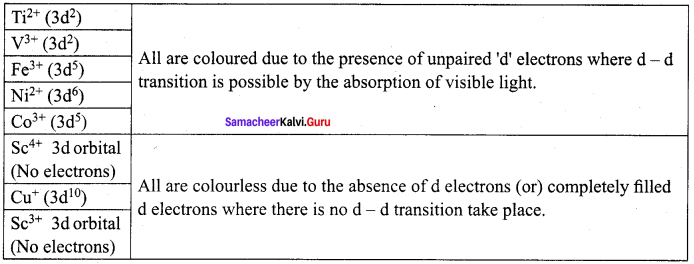Question 27.
Describe the variable oxidation state of 3d series elements.
1. The first transition metal Scandium exhibits only +3 oxidation state, but all other transition elements exhibit variable oxidation states by loosing electrons from (n-l)d orbital and ns orbital as the energy difference between them is very small.

2. At the beginning of the 3d series, +3 oxidation state is stable but towards the end +2 oxidation state becomes stable.

3. The number of oxidation states increases with the number of electrons available, and it decreases as the number of paired electrons increases. For example, in the 3d series, first element Sc has only one oxidation state +3 the middle element Mn has six different oxidation states from +2 to +7. The last element Cu shows +1 and +2 oxidation states only.

4. Mn2+ (3d5) is more stable than Mn4+ (3d3) is due to half filled stable configuration.

Question 28.
Which metal in the 3d series exhibits +1 oxidation state most frequently and why?
1. The first transition metal copper exhibits only +1 oxidation state.

2. It is unique in 3d series having a stable +1 oxidation state.
Cu (Z = 29) Electronic configuration is [Ar] 3d10 4s2

3. So copper element only can have +1 oxidation state.

Question 29.
Why first ionization enthalpy of chromium is lower than that of zinc?
The first ionization enthalpy of chromium is lower than that of zinc. Cr (Z = 24) Electronic configuration [Ar] 3d5 4s1. In the case of Cr, first electron has to be removed easily from 4s orbital to attain the more stable half filled configuration. So Cr has lower ionization enthalpy. But in the case of Zinc (Z = 30), electronic configuration [Ar] 3d10 4s2. The first electron has to be removed from the most stable fully filled electronic configuration becomes difficult and it requires more energy.

Question 30.
Transition metals show high melting points why?

1. All the transition metals are hard.
2. Most of them are hexagonal close packed, cubic close packed (or) body centered cubic which are characteristics of true metals.
3. The maximum melting point at about the middle of transition metal series indicates that d5 configuration of favourable for strong inter atomic attraction.
4. Due to the strong metallic bonds, atoms of the transition elements are closely packed and held together. This leads to high melting point and boiling point.

Samacheer Kalvi 12th Chemistry Transition and Inner Transition Elements Evaluate yourself

Question 1.
Compare the stability of Ni4+ and Pt4+ from their ionisation enthalpy values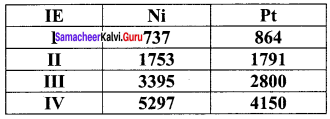Pt4+ compounds are stable than Ni4+ compounds because the energy needed to remove 4 electrons in Pt is less than that of Ni.

Question 2.
Why iron is more stable in +3 oxidation state than in +2 and the reverse is true for Manganese?
Fe (Z = 26). Electronic configuration [Ar] 3d4s2
Fe → Fe3+ + 3e
Fe3+ Electronic configuration p° [Ar]3d5.
If’d’ orbital is half filled, it is more stable than . Fe2+ where it is [Ar]3d6.
Mn (Z = 25). Electronic configuration [Ar]3d5 4s2
Mn → Mn2+ + 2e . By the loss of 2e, Mn2+ is more stable due to half filled configuration. Mn → Mn3+ + 3e.
Mn3+ Electronic configuration [Ar]3d4 4s°.
Among this Fe3+ is more stable than Fe2+ and the Mn2+ is more stable than Mn3+.

### Samacheer Kalvi 12th Chemistry Transition and Inner Transition Elements Additional Questions

Samacheer Kalvi 12th Chemistry Transition and Inner Transition Elements 1 Mark Questions and Answers

Question 1.
The elements whose atom has incomplete d sub-shell are called …………..
(a) s-block element
(b) Alkali metals
(c) transition elements
(d) Representative elements
(c) transition elements

2. Which one of the following is the other name of d-block elements?
(a) Chalcogens
(b) Halogens
(c) Inner-transition elements
(d) Transition elements
(d) Transition elements

Question 3.
Which metals play an important role in the development of human civilization?
(a) Al and Mg
(b) Na and K
(c) Fe and Cu
(d) Mn and Ni
(c) Fe and Cu

Question 4.
Which transition element is used in light bulb filaments?
(a) Al
(b) Ni
(c) W
(d) Fe
(c) W

Question 5.
Which metal is used in manufacturing artificial joints?
(a) Molybdenum
(6) Titanium
(c) Tungsten
(d) Iron
(b) Titanium

Question 6.
Which transition metal is applied in the manufacturing of boiler plants?
(a) Iron
(b) Copper
(c) Aluminium
(d) Molybdenum
(d) Molybdenum

Question 7.
Identify the transition metal present in Hemoglobin …………..
(a) Cobalt
(b) Iron
(c) Manganese
(d) Copper
(b) Iron

Question 8.
Which of the following transition metal is present in Vitamin B12?
(a) Cobalt
(b) Platinum
(c) Copper
(d) Iron
(a) Cobalt

Question 9.
The metal cobalt is present in
(a) Vitamin-A
(b) Vitamin-B
(c) Vitamin-B12
(d) Vitamin-B6
(c) Vitamin-B12

Question 10.
Consider the following statements.
(i) Transition metals occupy group-3 to group-12 of the modem periodic table.
(ii) Representative elements occupy group-3 to group-12 of the modem periodic table.
(iii) Except group-11 elements of all transition metals are hard.
(iv) d-block elements are mostly non-metals.

Which of the above statements is/are incorrect?
(a) (ii) and (iv)
(b) (i) and (iii)
(c) (iii) only
(d) (i) only
(a) (ii) and (iv)

Question 11.
Consider the following statements.
(i) d-block elements composed of 3d series, Sc to Zn (4th period).
(ii) 4d series composed of Y to Cd.
(iii) 5d series composed of La, Hf to Mercury.
(iv) d-block elements composed of 4d series Y to Cd.

Which of the above statements is/are incorrect
(a) (i) and (iv)
(b) (i), (ii) and (iii)
(c) (iii) and (iv)
(d) (iv) only
Ans.
(b) (i), (ii) and (iii)

Question 12.
Which of the following is the correct electronic configuration of Sc (Z = 21)?
(a) [Ar] 3d3
(b) [Ar] 3d’ 4s2
(c) [Ar] 3d2 4s1
(d) [Ar] 4s2 4p’
(b) [Ar] 3d’ 4s2

Question 13.
The correct electronic configuration of Cr is …………..
(a) [Ar] 3d4 4s2
(b) [Ar] 3d5
(c) [Ar] 3d5 4s1
(d) [Ar] 3d6
(c) [Ar] 3d5 4s1

Question 14.
Which of the following is the correct electronic configuration of copper?
(a) [Ar] 3d5 4s1
(b) [Ar] 3d10 4s1
(c) [Ar] 3d9 4s2
(d) [Ar] 3d8 4s2 4p1
(b) [Ar] 3d10 4s1

Question 15.
Which one of the following is the general electronic configuration of transition elements?
(a) [Noble gas] ns2 np6
(b) [Noble gas] ( n – 2 ) f1-14(n-l)d1-10 ns2
(c) [Noble gas] ( n – 1 ) d1-10 (n-l)f1-14 ns2
(d) [Noble gas] ( n – 1 ) d1-10 ns2
(d) [Noble gas] ( n – 1 ) d1-10 ns2

Question 16.
Which of the following d-block elements has the highest electrical conductivity at room temperature?
(a) Copper
(b) Silver
(c) Aluminium
(d) Tungsten
(b) Silver

Question 17.
Consider the following statements.
(i) The melting point decreases from Scandium to Vanadium in 3d series.
(ii) In 3d transition series, atomic radius decreases from Sc to V and upto copper atomic radius nearly remains the same.
(iii) As we move down in 3d transition series, atomic radius increases.

Which of the above statements is/are incorrect?
(a) (i) only
(b) (ii) only
(c) (iii) only
(d) (i), (ii) and (iii)
(a) (i) only

Question 18.
Which of the following transition element exhibit only +3 oxidation state?
(a) Cu
(b) Sc
(c) Mn
(d) Cr
(b) Sc

Question 19.
Which one of the following transition element has maximum oxidation states?
(a) Manganese
(b) Copper
(c) Scandium
(d) Titanium
(a) Manganese

Question 20.
Consider the following statements.
(i) In 3d series, the middle element Mn has +2 to +7 oxidation states.
(ii) The oxidation state of Ru and Os is +8.
(iii) Scandium has six different oxidation states.

Which of the above statements is/are not correct?
(a) (i) and (ii)
(b) (ii) only
(c) (i) only
(d) (iii) only
(d) (iii) only

Question 21.
Which one of the following elements show high positive electrode potential?
(a) Ti+
(b) Mn2+
(c) CO2+
(d) Cr2+
Ans.wer:
(c) CO2+

Question 22.
Which one of the following elements show high negative electrode potential?
(a) Copper
(b) Manganese
(c) Cobalt
(d) Zinc
(d) Zinc

Question 23.
Which one of the following is diamagnetic in nature?
(a) Ti3+
(b) Cu2+
(c) Zn2+
(d) V3+
(c) Zn2+

Question 24.
Which one of the following is paramagnetic in nature?
(a) Sc3+
(b) Ti4+
(c) V5+
(d) Cu2+
(d) Cu2+

Question 25.
Which of the following pair has maximum number of unpaired electrons?
(a) Mn2+, Fe3+
(b) CO3+, Fe2+
(c) Cr3+, Mn4+
(d) Ti2+, V3+
(a) Mn2+, Fe3+

Question 26.
Which of the following pair has d10 electrons?
(a) Ti3+, V4+
(b) CO3+, Fe2+
(c) Cu+ , Zn2+
(d) Mn2+, Fe3+
(c) Cu+, Zn2+

Question 27.
Which of the following is used as a catalyst in the manufacture of sulphuric acid form SO3.
(a) V3O5
(b) Rh-Ir
(c) Ni
(d) Fe
(a) V5O5

Question 28.
Which one of the following is Zeigler – Natta catalyst?
(a) CO2(CO)8
(b) Rh/Ir complex
(c) TiCl4 + Al(C2H5)3
(d) Fe / Mo
(c) TiCl4 + Al(C2H5)3

Question 29.
Which one of the following is used as a catalyst in the polymerisation of propylene?
(a) V2O5
(b) Pt
(c) TiCl4 + Al(C2H5)3
(d) Fe / Mo
(c)TiCl4 + Al(C2H5)3

Question 30.
Consider the following statements.
(i) Transition metal hydrides are used as powerful oxidising agents.
(ii) Metallic carbides are chemically active.
(iii) Interstitial compounds are hard and show electrical and thermal conductivity.

Which of the above statements is/are incorrect?
(a) (i) and (ii)
(b) (ii) and (iii)
(c) (iii) only
(d) (i) only
(a) (i) and (ii)

Question 31.
Which one of the following oxide is covalent?
(a) Cr2O3
(b) CrO
(c) Mn2O7
(d) Na2O
(c) Mn2O7

Question 32.
Which one of the following oxide is amphoteric in nature?
(a) CrO
(b) Cr2O3
(c) Mn2O7
(d) MnO
(b) Cr2O3

Question 33.
The oxidation state of Chromium in $${ CrO }_{ 4 }^{ 2- }$$ and in $${ Cr }_{ 2 }{ O }_{ 7 }^{ 2- }$$ are …………..
(a) +3, +6
(b) +7, +4
(c) +6, +6
(d) +4, +6
(c) +6, +6

Question 34.
Which one of the following is used to identify chloride ion in inorganic qualitative analysis?
(a) Barium chloride test
(b) Chromyl chloride test
(c) Brown ring test
(d) Ammonium molybdate test
(b) Chromyl chloride test

Question 35.
Which one of the following is the formula of chromyl chloride?
(a) CrOCl2
(b) CrCl3
(c) CrO2 Cl2
(d) CrCl
(c) CrO2 Cl2

Question 36.
Which ore is used to prepare potassium permanganate?
(a) Pyrolusite
(b) Chromite
(c) Argentite
(d) Cuprite
(a) Pyrolusite

Question 37.
Which one of the following geometry is possesed by permanganate ion?
(a) Pyramidal
(b) Tetrahedral
(c) Octahedral
(d) linear
(b) Tetrahedral

Question 38.
The hybridisation state of Mn+7 is permanganate ion is …………..
(a) sp2 hybridisation
(b) dsp2 hybridisation
(c) d2sp3 hybridisation
(d) sp3 hybridisation
(d) sp3 hybridisation

Question 39.
Which one of the following is known as Baeyer’s reagent?
(a) Cold dilute alkaline KMnO4
(b) Chromyl Chloride
(c) Acidified potassium dichromate
(d) Acidified potassium manganate
(a) Cold dilute alkaline KMnO4

Question 40.
Which reagent is used in the conversion of ethylene into ethylene glycol?
(a) Chromyl chloride
(b) Zeigler – Natta catalyst
(c) Cold dilute alkaline KMnO4
(d) Acidified K4Cr2O7
(c) Cold dilute alkaline KMnO4

Question 41.
Baeyer’s reagent is used to detect unsaturation in an organic compound.
(a) Chloride ion
(b) unsaturated organic compound
(c) Sulphate ion
(d) Chromate ion
(b) unsaturated organic compound

Question 42.
What is the equivalent weight of KMnO4 in acid medium?
(a) 158
(b) 52.67
(c) 31.6
(d) 392
(c) 31.6

Question 43.
What is the equivalent weight of KMnO4 in basic medium?
(a) 158
(b) 52.67
(c) 392
(d) 31.6
(a) 158

Question 44.
Which one of the following is used for the estimation of ferrous salts, oxalates, hydrogen peroxide and iodides?
(a) K2MnO4
(ft) KMnO4
(c) K2Cr2O7
(d) CrO2 Cl2
(b) KMnO4

Question 45.
Which of the following is the general electronic configuration of lanthanoids?
(a) [Xe] 4f7 3d1-10 5s2
(b) [Xe] 4f1-14 3d10 6s2
(c) [Xe] 5f2-14 4d10 6s2
(d) [Xe] 4f2-14 5d1-10 6s2
(d) [Xe] 4f2-14 5d1-10 6s2

Question 46.
The most common oxidation state of Lanthanoids is …………..
(a) + 4
(b) + 3
(c) + 6
(d) +5
(b) + 3

Question 47.
The expected electronic configuration of lanthanum (La) (Z = 57) is …………..
(a) [Xe] 4f1 5d° 6s2
(b) [Xe] 4f0 5d1 6s2
(c) [Xe] 4f3
(d) [Xe] 4f0 5d3
(a) [Xe] 4f1 5d° 6s2

Question 48.
The actual electronic configuration of La (Z = 57) is …………..
(a) [Xe] 4f1 5d° 6s2
(b) [Xe] 4f3
(c) [Xe] 4f0 5d1 6s2
(d) [Xe] 4f0 5d3
(c) [Xe] 4f0 5d1 6s2

Question 49.
Which of the following lanthanoids have half-filled 4f orbital?
(a) Gd
(b) Tb
(c) Lu
(d) La
(a) Gd

Question 50.
Which one of the following lanthanoids have completely filled 4f orbital?
(a) Gd and Eu
(b) La and Ce
(c) Yb and Lu
(d) Pr and Pm
(c) Yb and Lu

Question 51.
Which one of the following is the main cause of lanthanoid contraction?
(a) Poor shielding effect of 5f sub-shell
(b) More shielding effect of 4f sub-shell
(c) Poor shielding effect of 4f sub-shell
(d) More shielding effect of 5f sub-shell
(c) Poor shielding effect of 4f sub-shell

Question 52.
Which of the following pair has more or less same atomic radius due to lanthanide contraction?
(a) Ti and V
(b) Fm and Md
(c) No and Lr
(d) Zr and Hf
(d) Zr and Hf

Question 53.
Consider the following statement.
(i) All the actinoids are non radioactive.
(ii) Neptunium and other heavier elements are produced by artificial transformation of naturally occurring elements by nuclear reactions.
(iii) Most of the actinoids have long half lives.

Which of the above statements is/are not correct.
(a) (i) only
(b) (i) and (ii)
(c) (ii) and (iii)
(d) (i) and (iii)
(d) (i) and (iii)

Question 54.
The general valence shell electronic configuration of actinoids is …………..
(a) [Xe] 4f2-14 5d0-2 6s2
(b) [Rn] 4f2-14 5d0-2 6s2
(c) [Rn] 5f2-14 6d0-1 7s2
(d) [Rn] 4f0-7 5d0-1 s2
(c) [Rn] 5f2-14 6d0-2 7s2

Question 55.
Which pair of actinoids show +2 oxidation state?
(a) Am and Th
(b) Pa and U
(c) Pu and Cm
(d) No and Lr
(a) Am and Th

Question 56.
Neptunium and Plutonium exhibit the maximum oxidation state as …………..
(a) + 8
(b) + 7
(c) + 6
(d) + 4
(b) + 7

Question 57.
Consider the following statement.
(i) Most of the actinoids are coloured.
(ii) Actinoids show greater tendency to form complexes.
(iii) Most of the actinoids are non-radioactive.

Which of the above statements is/are correct.
(a) (i) only
(b) (i) and (iii)
(c) (i) and (ii)
(d) (ii) and (iii)
(c) (i) and (ii)

Question 58.
Consider the following statement.
(i) Lanthanoids do not form oxo cations.
(ii) Most of the lanthanoids are colourless.
(iii) Binding energy of 4f orbitals are lower.

Which of the above statement is/are not correct.
(a) (i) and (ii)
(b) (iii) only
(c) (i) and (iii)
(d) (i), (ii) and (iii)
(b) (iii) only

Question 59.
Which one of the following is more basic in nature?
(a) La(OH)3
(b) Ce(OH)3
(c) Gd(OH)3
(d) Lu(OH)3
(a) La(OH)3

Question 60.
Which one of the following is less basic in nature?
(a) La(OH)3
(b) Gd(OH)3
(c) Lu(OH)3
(d) Ce(OH)3
(c) LU(OH)3

II. Fill in the blanks.

1. Transition elements occupy the central position of the periodic table between …………. elements.
2. Except …………. elements, all transition metals are hard and have very high melting point.
3. The metal present in Vitamin – B12 is ………….
4. …………. metal is used in manufacture of artificial joints.
5. The extra stability of Cr and Cu is due to …………. of electrons and exchange energy.
6. Of all the known elements …………. has the highest electrical conductivity at room temperature.
7. The maximum melting point at about the middle of transition metal series indicates that …………. configuration is favourable for strong attraction.
8. The atomic radius of 5d elements and 4d elements are nearly same due to ………….
9. Ni (II) compounds are thermodynamically …………. than Pt (II) compounds.
10. The first transition metal …………. exhibits only +3 oxidation state.
11. The middle transition element …………. has six different oxidation states.
12. The oxidation state of Ru and Os is ………….
13. …………. is unique in 3d series having a stable +1 oxidation state.
14. The substance which is oxidised is a …………. agent and the one which is reduced is an …………. agent.
15. The oxidising and reducing power of an element is measured in terms of ………….
16. If the E° of a metal is large and negative, the metal is a ………….
17. The species with all paired electrons exhibit ………….
18. The magnetic moment of an ion is given by ………….
19. Many industrial processes use …………. or their as catalyst.
20. In the catalytic hydrogenation of an alkene …………. is used as catalyst.
21. In the preparation of acetic acid from acetaldehyde the catalyst used in ………….
22. The catalyst used in the hydroformylation of olefins is ………….
23. …………. catalyst is used in polymerization of propylene.
24. Metallic carbides are chemically ………….
25. Except Scandium all other 3d series transition elements form …………. metal oxides.
26. Cr2O3 …………. is and CrO is …………. in nature.
27. Mn2O7 dissolves in water to give ………….
28. On heating potassium dichromate, it decomposes to give …………. and molecular oxygen.
29. Potassium dichromate is a powerful …………. agent in acidic medium.
30. ………….is used in leather tanneries for chrome tanning.
31. Potassium dichromate is used in quantitative analysis for the estimation of …………. and ………….
32. Permanganate ion has …………. geometry in which Mn+7 is …………. hybridised.
33. Cold dilute alkaline KMnO4 is known as ………….
34. …………. is used for the treatment of skin infections and fungal infections of the foot.
35. Baeyer’s reagent is used for detecting …………. in an organic compounds.
36. The equivalent weight of KMnO4 in neutral medium is ………….
37. The common oxidation state of lanthanoids is ………….
38. Due to the decrease in the size of Ln3+ ions, the ionic character of Ln – OH bond decreases which results in the ………….
39.  All the actinoids are …………. and most of them have …………. half lives.
40. ………….do not form oxo cations.

1. sandpblock
2. group-II
3. cobalt
4. Titanium
5. symmetrical distribution
6. silver
7. d5, inter atomic
8. lanthanoid contraction
9. more stable
10. Scandium
11. Manganese
12. + 8
13. Copper
14. reducing, oxidising
15. Standard electrode potential
16. powerful reducing agent
17. diamagnetism
18. μ=$$g\sqrt { S(S+1) }$$μB
19. transition metals, compounds
20. Nickel
21. Rh/Ir complex
22. CO2(CO)8
23. Zeigler – Natta (or) TiCl4 + Al(C2H5)3
24. inert
25. ionic
26. amphoteric, basic
27. permanganic acid (HMnO4)
28. Chromium (III) oxide – Cr2O3
29. Potassium dichrom.ate
30. iron compounds, iodides
31. tetrahedral,sp3
32. Baeyer’s reagent
33. Potassium permanganate
34. unsaturation
35. 52.67
36. + 3
37. decrease in the basicity
39. Lanthanoids

III. Match the following using the code given below.

Question 1.
A. Tungsten – 1. Development of human civilization
B. Titanium – 2. Light bulb filament
C. Molybdenum – 3. Artificial joint
D. Copper – 4. Boiler plants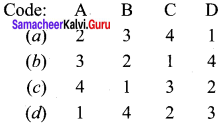(a) 2, 3, 4, 1

Question 2.
A. Iron – 1. Artificial joints
B. Platinum – 2. Hemoglobin
C. Cobalt – 3. Catalysis
D. Titanium – 4. Vitamin – B12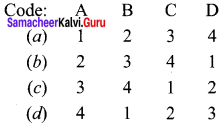(b) 2, 3, 4, 1

Question 3.
A. Sc to Zn – 1. 5d series
B. Y to Cd – 2. Actinoids
C. LatoHg – 3. 3dseries
D. Ac to Lr – 4. 4d series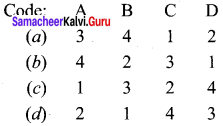(a) 3, 4, 1, 2

Question 4.
A. Cr – 1. [Ar] 3d10 4s2
B. Cu – 2. [Ar] 3d5 4s1
C. Zn – 3. [Ar]3d1 4s2
D. Sc – 4. [Ar] 3d10 4s1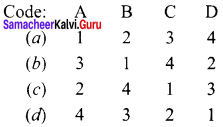(c) 2, 4, 1, 3

Question 5.
A. Scandium – 1. + 7
B. Manganese – 2. + 2
C. Copper – 3.+3
D. Titanium – 4. + I(a) 3, 1, 4, 2

Question 6.
A. Sc3+ – 1. 3d1
B. Ti3 – 2. 3d0
C. Mn2+ – 3. 3d10
D. Zn2+ – 4. 3d5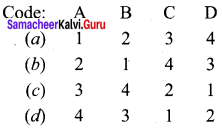(b) 2, 1, 4, 3

IV. Assertion and Reason.

Question 1.
Assertion (A) – Cr and Cu having [Ar] 3d5 4s1 and [Ar] 3d10 4s1 are more stable.
Reason (R) – The extra stability of elements Cr and Cu is due to symmetrical distribution of electrons and exchange energy.
(a) Both (A) and (R) are correct and (R) explains (A).
(b) Both (A) and (R) are correct but (R) is not the correct explanation of (A).
(c) (A) is correct but (R) is wrong.
(d) (A) is wrong but (R) is correct.
(a) Both (A) and (R) are correct and (R) explains (A).

Question 2.
Assertion (A) – In 3d transition elements, the expected decrease in atomic radius is observed from Sc to V, thereafter upto Cu, the atomic radius nearly remains the same.
Reason (R) – As we move from Sc to V, the added 3d electrons only partially shield the increased nuclear charge but upto Cu, the extra electron added to 3d sub-shell repel the 4s electrons and the slight increase in nuclear charge operated in opposite direction and it leads to constancy in atomic radii.
(a) Both (A) and (R) are correct and (R) explains (A).
(b) Both (A) and (R) are correct but (R) is not the correct explanation of (A).
(c) (A) is correct but (R) is wrong.
(d) (A) is wrong but (R) is correct.
(a) Both (A) and (R) are correct and (R) explains (A).

Question 3.
Assertion (A) – In transition metal series, the ionization enthalpy increases.
Reason (R) – This is due to increase in nuclear charge corresponding to the filling of d electrons.
(a) Both (A) and (R) are correct and (R) explains (A).
(b) Both (A) and (R) are correct but (R) is not the correct explanation of (A).
(c) (A) is correct but (R) is wrong.
(d) (A) is wrong but (R) is correct.
(a) Both (A) and (R) are correct and (R) explains (A).

Question 4.
Assertion (A) – Ni (II) compounds are thermodynamically more stable than Pt (II) compounds.
Reason (R) – The energy required to form Ni2- is less than that of Pt2+.
(a) Both (A) and (R) are correct and (R) explains (A).
(b) Both (A) and (R) are correct but (R) is not the correct explanation of (A).
(c) (A) is correct but (R) is wrong.
(d) (A) is wrong but (R) is correct.
(a) Both (A) and (R) are correct and (R) explains (A).

Question 5.
Assertion (A) – Except Scandium all 3d series, transition elements exhibit variable oxidation states.
Reason (R) – By loosing electrons from (n – l)d orbital and ns orbital as the energy difference between them is very small.
(a) Both (A) and (R) are correct and (R) explains (A).
(b) Both (A) and (R) are correct but (R) is not the correct explanation of (A).
(c) (A) is correct but (R) is wrong.
(d) (A) is wrong but (R) is correct.
(a) Both (A) and (R) are correct and (R) explains (A).

Question 6.
Assertion (A) – Mn2+ is more stable than Mn4+.
Reason (R) – Mn2+(3d5) is more stable than Mn4+(3d3) is due to extra stability of half-filled electronic configuration.
(a) Both (A) and (R) are correct and (R) is the correct explanation of (A)
(b) Both (A) and (R) are correct but (R) is not the correct explanation of (A)
(c) (A) is correct but (R) is wrong
(d) (A) is wrong but (R) is correct
(a) Both (A) and (R) are correct and (R) is the correct explanation of (A)

Question 7.
Assertion (A) – Copper is unique in 3d series having a stable +1 oxidation state.
Reason (R) – Copper is prone to disproportionate to the +2 and 0 oxidation states.
(a) Both (A) and (R) are correct but (R) is not the correct explanation of (A).
(b) Both (A) and (R) are correct and (R) explains (A).
(c) (A) is correct but (R) is wrong.
(d) (A) is wrong but (R) is correct.
(b) Both (A) and (R) are correct and (R) explains (A).

Question 8.
Assertion (A) – Transition metals form large number of complexes.
Reason (R) – Transition metals are small and highly charged and they have vacant low energy orbitals to accept an electron pair donated by other groups.
(a) Both (A) and (R) are correct and (R) is the correct explanation of (A)
(b) Both (A) and (R) are correct but (R) is not the correct explanation of (A)
(c) (A) is correct but (R) is wrong
(d) (A) is wrong but (R) is wrong
(a) Both (A) and (R) are correct and (R) is the correct explanation

V. Find the odd one out.

Question 1.
(a) Sc
(b) Ti
(c) Y
(d) Cr
(c) Y
Reason: Yttrium be1ons to 4d series whereas others are 3d series

Question 2.
(a)Ru
(b)Rh
(c)Pd
(d) Pt
(d) Pt
Reason: Pt belongs to 5d series whereas others are 4d series.

Question 3.
(a) Th
(b) La
(c) Ce
(d) Lu
(a) Th
Reason: Th belongs to actinoids whereas others are lanthanoids.

Question 4.
(a) La
(b) Pr
(c) Am
(d) Lu
(c) Am
Reason: Am belongs to actinoids whereas others are lanthanoids.

Question 5.
(a) Ce
(b) Th
(c) U
(d) Pu
(a) Ce
Reason: Ce belongs to lanthanoids whereas others are actinoids.

Question 6.
(a) Ac
(b) U
(c) Pa
(4) Np
(a) Ac
Reason: Except Actinium all the remaining elements are synthetically prepared.

Question 7.
(a) Ti2+
(b) V2+
(c) Zn2+
(d) Cu2+
(d) Cu2+
Reason: It has positive reduction potential whereas others have negative reduction potential.

Question 8.
(a) CO3+
(b) Cr3+
(c) V3+
(4) Ti3+
(a) CO3+
Reason: It has positive reduction potential whereas others have negative reduction potential.

Question 9.
(a) Mn3+
(b) Fe3+
(c) Cr3+
(d) CO3+
(c) Cr3+
Reason: It has negative reduction potential whereas others have positive reduction potential.

Question 10.
(a) SC3+
(b) Ti4+
(c) V5+
(d) Cu2+
(d) Cu2+
Reason: It is paramagnetic whereas others are diamagnetic.

Question 11.
(a) Cr3+
(b) Mn4+
(c) V2+
(d) Zn2+
(d) Zn2+
Reason: It is diamagnetic whereas others are paramagnetic

VI. Find Out the correct pair.

Question 1.
(a) $${ CrO }_{ 4 }^{ – }$$ and Cr2$${ O }_{ 7 }^{ 2- }$$
(b) $${ MnO }_{ 4 }^{ – }$$ and $${ MnO }_{ 4 }^{ 2- }$$
(c) H2CrO4 and HMnO4
(d) Cr2O3 and Mn2O7
(a) $${ CrO }_{ 4 }^{ – }$$ and Cr2$${ O }_{ 7 }^{ 2- }$$
In this pair Cr has +6 oxidation states whereas in others the metal has different oxidation state.

Question 2.
(a) Zn, Cu
(b) Hf, Zr
(c) Ag , Au
(d) Ti, Cu
(b) Hf, Zr
This pair has same atomic radius whereas others have different atomic radius.

Question 3.
(a) Ru , Os
(b) Mn , Cu
(c) Sc , Cu
(d) Ni, Co
(a) Ru , Os
Both has +8 as oxidation state whereas others have different oxidation state.

Question 4.
(a) Ti2+, CO2+
(b) Cr2+, Mn3+
(c) Fe2+ and CO3+
(d) CO3+ and Cu2+
(d) CO3+ and Cu2+
Both have positive electrode potential whereas others have different value.

Question 5.
(a) Cu2+, Zn2+
(b) CO3+, Cr3+
(c) Ti3+, V3+
(d) Mn3+, Cr3+
(c) Ti3+, V3+
have negative electrode potential whereas others have different values.

VII. Find out the incorrect pair.

Question 1.
(a) Sc3+, Ti4+
(b) Ti3+, Ti2+
(c) Cr2+, Mn3+
(d) Cu+, Zn2+
(b) Ti3+, Ti2+
have d1 and d1 configuration whereas others have same configuration.

Question 2.
(a) Sc and Zn
(b) Y and Cd
(c) Ag and Au
(d) Na and K
(d) Na and K. They belong to alkaline metals whereas others are d-block elements.

Samacheer Kalvi 12th Chemistry Transition and Inner Transition Elements 2 Mark Questions and Answers

Question 1.
d-block elements are called transition elements. Justify this statement.
1. d-block elements occupy the central position of the periodic table, between s and p block elements.

2. Their properties are transitional between highly reactive metals of s-block and elements of p-block which are mostly non-metals. That is why d-block elements are called transitional elements.

Question 2.
How many series are in d-bloclc elements? What are they?

• There are 4 series in d-block e1ements They are,
• 3d series – 4th period – Scandium to Zinc
• 4d series – 5th period – Yttrium to Cadmium
• 5d series – 6th period – Lanthanum to Mercury
• 6d series – 7th period – Actinium to Californium

Question 3.
Zn, Cd, Hg belong to d-block elements even though they do not have partially filled d-orbitals. Give reason.

1. Zn, Cd, Hg belong to d-block elements even though they do not have partially filled d-orbitals either in their elemental state or in their normal oxidation states.
2. However they are treated as transition elements, because their properties are an extension of the properties of the respective transition elements.

Question 4.
Applying Aufbau principle, write down the electronic configuration of Sc (Z = 21) and Zn (Z = 30).

1. According to Aufbau principle, the electron first fills the 4s orbital before 3d orbital.
2. Sc (Z = 21) Is2 2s2 2p6 3s2 3p6 4s2 3d1
• Zn (Z = 30) Is2 2s2 2p6 3s2 3p6 4s2 3d10

Question 5.
At the end of 3d series, d-orbitals of Zinc contain 10 electrons in which the repulsive interaction between the electrons is more than the effective nuclear charge and hence the orbitals slightly expand and atomic radius slightly increases.

Question 6.
Write a not about oxidation state of 3d series.

1. The number of oxidation states increases with the number of electrons available, and it decreases as the number of paired electrons increases.
2. Hence, the first and last elements show less number of oxidation states and the middle elements with more number of oxidation states.
3. For example, the first element Sc has only one oxidation state +3, the middle element Mn has six different oxidation states from +2 to +7. The last element Cu shows +1 and +2 oxidation states only.

Question 7.
Mn2+ is more stable than Mn4+. Why?

1. The relative stability of different oxidation states of 3d metals is correlated with the extra stability of half-filled and fully filled electronic configurations.
2. Example – Mn2+ (3d5) is more stable than Mn4+ (3d3)

Question 8.
Ru and Os have highest oxidation state in which compounds? Explain with example.

1. Ru and Os have +8 as the highest oxidation state.
2. The highest oxidation state of 4d and 5d elements are found in their compounds with the higher electronegative elements like O, F and Cl. For example: RuO4, OsO4

Question 9.
Copper is unique in 3d series. Prove this statement.
Copper is unique in 3d series having a stable +1 oxidation state. It is prone to disproportionate to the +2 and 0 oxidation states.

Question 10.
Define – Standard electrode potential.
Standard electrode potential is the value of the standard emf of a cell in which molecular hydrogen under standard pressure (latm) and temperature (273K) is oxidised to solvated protons at the electrode.

Question 11.
Which metal is used to reduce Cr3+ ion? Why?
A stable Cr3+ ion, strong reducing agent which has high negative value for reduction potential like metallic zinc (E° = – 0.76 V) is required. Metallic zinc is a powerful reducing agent due to its large negative values.

Question 12.
Sc3+, Ti4+, V5+ are diamagnetic. Give reason.

1. Sc3+, Ti4+, V5+ have d° electronic configuration, n = 0
2. µ = $$\sqrt { 0(0+2) }$$ = 0 µB So they are diamagnetic.

Question 13.
Calculate the magnetic moment of Ti3+ and V4+.
Ti (Z = 22) Ti3+ 3d1
V (Z = 23) V4+ 3d1
µ = $$\sqrt { 1(1+2) }$$ = $$\sqrt { 35 }$$ = 1.73 µB. So they are paramagnetic.

Question 14.
Cr3+, Mn4+, V2+ are paramagnetic. Calculate their magnetic moment values.
Cr3+, Mn4+, V2+ Configuration is d3. Due to 3 unpaired electrons, they are paramagnetic.  µ = $$\sqrt { 3(3+2) }$$ = $$\sqrt { 35 }$$ = 3.87 µB

Question 15.
Mn2+, Fe3+ have high magnetic moment. Prove it.

1. Mn2+, Fe3+ configuration is d5.
2. µ = $$\sqrt { 5(5+2) }$$ = $$\sqrt { 35 }$$ = 5.916 µB
Among 3d series, Mn2+, Fe3+ have high magnetic moment as 5.916 µB .

Question 16.
How many unpaired electrons are present in CO3+, Fe2+? Calculate their magnetic moment.
CO (Z = 27) CO3+ [Ar] 3d6
Fe (Z = 26) Fe2+ [Ar] 3d6
The number of unpaired electrons are 4 as follows: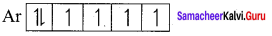Their magnetic moment is µ = $$\sqrt { 4(4+2) }$$ = $$\sqrt { 24 }$$ = 4.89 µB

Question 17.
Calculate the magnetic moment and the number of unpaired electrons in Cu2+.
Cu (Z = 29) Electronic configuration [Ar] 3d10 4s1
Cu2+ Electronic configuration [Ar] 3d9
The number of unpaired electrons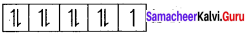is 1.
Magnetic moment µ = $$\sqrt { 1(1+2) }$$ = $$\sqrt { 3 }$$ = 1.732 µB

Question 18.
Cu+, Zn2+ are diamagnetic. Prove it.
Cu+, Zn2+ electronic configuration [Ar] 3d10
The number of unpaired electron is 0.
µ = $$\sqrt { 0(0+2) }$$ = 0 µB. Cu+, Zn2+ are diamagnetic.

Question 19.
Most of the transition metals act as catalyst. Justify this statement.
1. Many industrial processes use transition metals or their compounds as catalysts. Transition metal has energetically available d orbitals that can accept electrons from reactant molecule or metal can form bond with reactant molecule using its ‘d’ electrons.

2. For example, in the catalytic hydrogenation of an alkene, the alkene bonds to an active site by using its n electrons with an empty d orbital of the catalyst.

Question 20.
Explain the catalytic hydrogenation of alkene to alkane with equation.
The σ bond in the hydrogen molecule breaks, and each hydrogen atom forms a bond with a d electron on an atom in the catalyst Nickel. The two hydrogen atoms then bond with the partially broken π -bond in the alkene to form an alkane.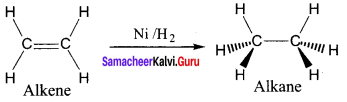Question 21.
Which catalyst is used in the hydroformylation of olefins? Give equation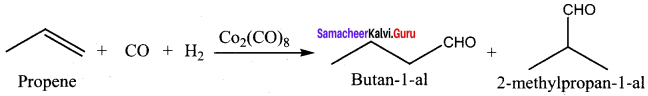Question 22.
Which catalyst is used in the conversion of acetaldehyde to acetic acid? Give equation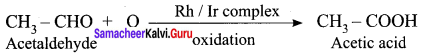Question 23.
What is Zeigler -Natta catalyst? In which reaction it is used? Give equation.
A mixture of TiCl4 and trialkyl aluminium is Zeigler – Natta catalyst. It is used in the polymerization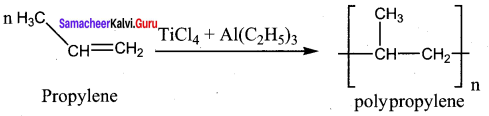Question 24.
d-block elements readily form complexes. Give reason.
1. Transition elements (d-block elements) have a tendency to form coordination compounds (complexes) with a species that has an ability to donate an electron pair to form a coordinate

2. Transition metal ions are small and highly charged and they have vacant low energy orbitals to accept an electron pair donated by other groups. Due to these properties, transition metals form large number of complexes.

3. Examples – [Fe(CN)6]4-, [CO(NH3)6]3+

Question 25.
Prove that acidified potassium dichromate is a powerful oxidising agent.
K2Cr2O7 act as power oxidising agent in acidic medium. In the presence of H+ ions, the oxidation state of Cr froms Cr6+ is changed to Cr3+
Cr2$${ O }_{ 7 }^{ 2- }$$ + 14H+ + 6 → 2Cr3+ + 6Fe3+ + 7H2O

Example:
Acidified K2Cr2O7 oxidises Ferrous salts to Ferric salts.
Cr2$${ O }_{ 7 }^{ 2- }$$ + 6Fe2+ + 14H+ → 2Cr3+ + 6Fe3+ + 7H2O.

Question 26.
What are the uses of potassium dichromate?
K2Cr2O7 is used

1. as a strong oxidising agent
2. in dyeing and printing
3. in leather tanneries for chrome plating
4. in quantitative analysis for the estimation of iron compounds and iodides

Question 27.
Draw and explain about the structure of permanganate ion.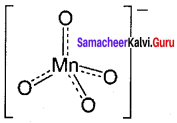Permanganate ion has tetrahedral geometry in which the central Mn7+ is sp3 hybridised.

Question 28.
Explain the action of heat on potassium permanganate.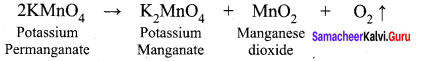Question 30.
What happens when thiosuiphate ion is treated with permanganate ion?
Permanganate ion oxidises thiosulphate into sulphate.
$${ 8MnO }_{ 4 }^{ – }$$ + 3S2$${ O }_{ 3 }^{ 2- }$$ → $${ 6SO }_{ 4 }^{ 2- }$$ + 8MnO2 + 2OH

Question 31.
What is Baeyer’s reagent? Where it is used?

1. Cold dilute alkaline KMnO4 is known as Baeyer’s reagent. It is used to oxidise alkene into diols.
2. For example, ethylene can be converted into ethylene glycol and this reaction is used as a test for unsaturation.

Question 32.
Acidified KMnO4 is a very strong oxidising agent. Prove it.
1. In the presence of dilute sulphuric acid, potassium permanganate acts as a very strong oxidising agent. Permanganate ion is converted into Mn2+ ion.
$${ MnO }_{ 4 }^{ – }$$ + 8H+ + 5e → Mn2+ + 4H2O

2. Permanganate oxidises ferrous salt to ferric salt.
$${ 2MnO }_{ 4 }^{ – }$$ + 10Fe2+ + 16H+ → 2Mn2+ + 10Fe3+ + 8H2O

Question 33.
KMnO4 does not act as oxidising agent in the presence of HCl. Why?
HCl cannot be used for making acidified KMnO4 as oxidising agent, since it reacts with KMnO4 as follows.
$${ 2MnO }_{ 4 }^{ – }$$ + 10Cl + 16H+ → 2Mn2+ + 5Cl2 + 8H2O

Question 34.
HNO3 cannot be used as an acid medium along with KMnO4. Why?
HNO3 cannot be used since it is a good oxidising agent and it reacts with reducing agents in the reaction.

Question 35.
Among HCl, HNO3 and H2SO4, which is the suitable medium for KMnO4 in oxidising reaction?
HCl and HNO3 cannot be used. HCl react with KMnO4. HNO3 is itself a good oxidising agent. However, H2SO4 is found to be most suitable since it does not react with potassium permanganate.

Question 36.
Explain about the causes of lanthanide contraction.
1. As we move from one element to another in 4f series ( Ce to Lu) the nuclear charge increases by one unit and an additional electron is added into the same inner 4f sub-shell.

2. 4f sub-shell have a diffused shapes and therefore the shielding effect of 4f electrons are relatively poor. Hence, with increase of nuclear charge, the valence shell is pulled slightly towards nucleus.

3. As a result, the effective nuclear charge experienced by the 4f elelctoms increases and the size of Ln3+ ions decreases.

Samacheer Kalvi 12th Chemistry Transition and Inner Transition Elements 3 Mark Questions and Answers

Question 1.
Cr and Cu are more stable. Give reason.
Answwer:
1. The electronic configuration of Cr and Cu are [Ar] 3d3 4s1 and [Ar] 3d10 4s1 respectively. The extra stability of half filled and fully filled d orbitals, as already explained in XI STD, is due to symmetrical distribution of electrons and exchange energy.

2. The extra stability of half filled and fully filled d orbitals is due to symmetrical distribution of electrons and exchange energy.

3. When the d orbitals are considered together, they will constitute a sphere. So the half filled and fully filled configuration leads to complete symmetrical distribution of electron density.

4. On the other hand, an unsymmetrical distribution of electron density will result in building up of a potential difference. To decrease this and to achieve a tension free state with lower energy, a symmetrical distribution is preferred.

Question 2.
Explain about the metallic behaviour of d-block elements.
1. All the transition elements are metals. They are good conductors of heat and electricity. Of all the known elements, silver has the highest electrical conductivity at room temperature.

2. Most of the transition elements are hexagonal close packed, cubic close packed or body centered cubic which are the characteristics of true metals.

Question 3.
Explain about the variation of melting point among the transition metal series.
1. As we move from left to right along the transition metal series, melting point first increases as the number of unpaired d electrons available for metallic bonding increases, reach a maximum value and then decreases, as the d electron pairs up and become less available for bonding.

2. For example, in the first series the melting point increases from Scandium to a maximum of 2183 K for Vanadium, which is close to 2180K for chromium.

3. Manganese in 3d series and has low melting point. The maximum melting point at about the middle of transition metal series indicates that d5 configuration is favorable for strong interatomic attraction.

Question 4.
Explain about the variation of atomic radius along a period of 3d series.
1. In general, atomic radius decreases along a period. But for the 3d transition elements, the expected decrease in atomic radius is observed from Sc to V , thereafter upto Cu the atomic radius nearly remains the same.

2. As we move from Sc to Zn in 3d series, the extra electrons are added to the 3d orbitals, the added 3d electrons only partially shield the increased nuclear charge and hence the effective nuclear charge increases slightly.

3. However, the extra electrons added to the 3d sub shell strongly repel the 4s electrons and these two forces are operated in opposite direction and as they tend to balance each other, it leads to constancy in atomic radii.

Question 5.
Ni (II) compounds are more stable than Pt (II) compounds. Give reason.
1. The ionisation enthalpy values can be used to predict the thermodynamic stability of their compounds. .

2. For Nickel I.E1 + I.E2 = 737+ 1753
= 2490 kJ mol-1
For platinum, I.E1 + I.E2 = 864+ 1791
= 2655 kJ mol-1
Since, the energy required to form Ni2+ is less than that of Pt2+ , Ni(II) compounds are thermodynamically more stable than Pt(II) compounds.

Question 6.
Compare the reduction potentials of Mn3+ / Mn2+ and Fe3+ / Fe2+ .
1. Mn3+ + e → Mn2+
E° = + 1.51V
Fe3+ + e → Fe2+
E° = + 0.77V

2. The high reduction potential of Mn3+ / Mn2+ indicates Mn2+ is more stable than Mn3+. For Fe3+ / Fe2+ the reduction potential is 0.77V, this low value indicates that both Fe3+ and Fe2+ can exist under normal conditions.

3. The drop from Mn to Fe is due to the electronic structure of the ions concerned. Mn3+ has 3d4 configuration while that of Mn2+ is 3d5. The extra stability associated with a half filled d sub-shell makes the reduction of Mn3+ very feasible.

Question 7.
How alloys are formed in d-biock elements?
1. An alloy is formed by blending a metal with one or more other elements. The elements may be metals or non-metals or both.

2. The bulk metal is named as solvent, and the other elements in smaller portion is called solute.

3. According to Hume – Rothery rule to form an alloy, the difference between the atomic radii of the solvent and solute is less than 15%. Both the solvent and solute must have the same crystal structure and valence and their electro negativity difference must be close to zero.

4. Since their atomic sizes are similar and one metal atom can be easily replaced by another metal atom from its crystal lattice to form an alloy. The alloys are hard and have high melting points. Examples – Gold – copper alloy.

Question 8.
What are interstitial compounds? Give their properties.
An interstitial compound or alloy is a compound that is formed when small atoms like hydrogen, boron, carbon or nitrogen are trapped in the interstitial holes in a metal lattice. They are usually non-stoichiometric compounds, e.g., TiC, ZrH1.92, Mn4N.

1. They are hard and show electrical and thermal conductivity.
2. They have high melting points.
3. Transition metal hydrides are used as powerful reducing agents.
4. Metallic carbides are chemically inert.

Question 9.
Explain the action of heat on potassium dichromate.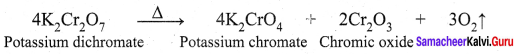Question10.
Draw and explain about the structure of chromate and dichromate ion.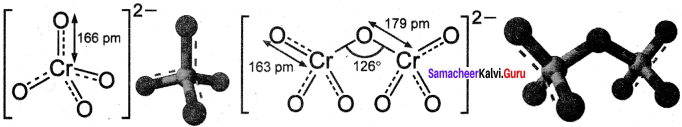1. Both chromate and dichromate ions are oxo anions of chromium and they are moderately strong oxidising agents.
2. In both structures, chromium is in +6 oxidation state.
3. In an aqueous solution, chromate and dichromate ions can be inter convertible, and in an alkaline solution, chromate ion is predominant, whereas dichromate ion becomes predominant in acidic solutions. .

Question 11.
Explain the action of acidified K2Cr2O7 – with

1. Iodide
2. Sulphide

1. Acidified K2Cr2O7 oxidises iodide ions to iodine.
Cr2$${ O }_{ 7 }^{ 2- }$$ + 6I + 14H+ → 2Cr3+ +3I2 + 7H2O

2. Acidified K2Cr2O7 oxidises Sulphide ions to Sulphur.
Cr2$${ O }_{ 7 }^{ 2- }$$ + 3S2- + 14H+ → 2Cr3+ + 3S + 7H2O

Question 12.
Explain the action of acidified K2Cr2O7 with

1. Sulphur dioxide
2. Alcohols.

1. Acidified K2Cr2O7 oxidises SO2 to sulphate ion.
Cr2$${ O }_{ 7 }^{ 2- }$$ + 3SO2 + 2H+ -> 2Cr3+ + $${ 3SO }_{ 4 }^{ 2- }$$ + H2O

2. Acidified K2Cr2O7 oxidises alcohol to acid. .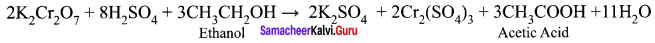Question 13.
1. When potassium dichromate is heated with any chloride salt in the presence of Conc.H2SO4, orange red vapours of chromyl chloride (CrO2Cl2) is evolved. This reaction is used to confirm the presence of chloride ion in inorganic qualitative analysis.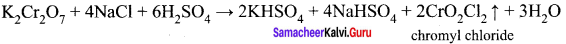2. The chromyl chloride vapours are dissolved in sodium hydroxide solution and then acidified with acetic acid and treated with lead acetate. A yellow precipitate of lead chromate is obtained.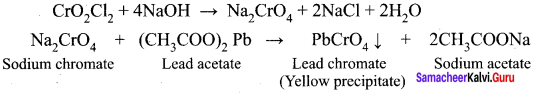Question 14.
Explain the action of Conc.H2SO4 on potassium permanganate.
1. On treating with cold conc.H2SO4, it decomposes to form manganese heptoxide, which subsequently decomposes explosively.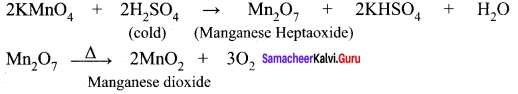2. With hot Conc.H2SO4, potassium permanganate give MnSO4 [Manganese(II) sulphate]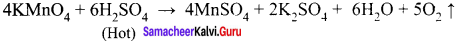Question 15.
What are the uses of Potassium permanganate?
Potassium permanganate is used,

1. as a strong oxidising agent.
2. for the treatment of various skin infections and fungal infections of foot.
3. used in water treatment industries to remove iron and hydrogen sulphide from well water.
4. as a Baeyer’s reagent for detecting unsaturation in an organic compound.
5. in quantitative analysis for the estimation of ferrous salts, oxalates, hydrogen peroxide (H2O2) and iodides.

Question 16.
Calculate the equivalent weight of KMnO4 in

1. Acidic medium
2. Basic medium
3. Neutral medium

1. Equivalent weight of KMnO4 in acidic medium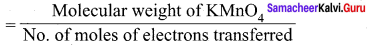2. Equivalent weight of KMnO4 in basic medium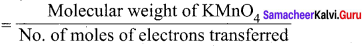3. Equivalent weight of KMnO4 in neutral medium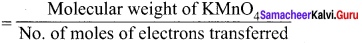Question 17.
Explain about the oxidation state of actinoids.

1. The most common oxidation state of actinoids is +3.
2. In addition to that actinoids show variable oxidation states such as +2, +3, +4, +5, +6 and +7.
3. The elements Americium (Am) and Thorium (Th) show +2 oxidation state in some compounds.
4. Th, Pa, U, Np, Pu and Am show +5 oxidation states.
5. Np and Pu exhibit +7 oxidation state.

Question 18.
Write the electronic configuration of

1. Ac (Z = 89)
2. Am (Z = 95)
3. Lr (Z = 103)

1. Ac (Z = 89): [Rn] 5f0 6d1 7s2
2. Am (Z = 95) : [Rn] 5f7 6d0 7s2
3. Lr (Z = 103): [Rn] 5f14 6d1 7s2

Samacheer Kalvi 12th Chemistry Transition and Inner Transition Elements 5 Mark Questions and Answers

Question 1.
Explain about the magnetic properties of transition elements.
1. Most of the compounds of transition elements are paramagnetic.

2. Materials with no elementary magnetic dipoles are diamagnetic. In other words a species with all paired electrons exhibits diamagnetism.

3. Paramagnetic solids having unpaired electrons possess magnetic dipoles which are isolated from one another.

4. Ferromagnetic materials have domain structure and in each domain the magnetic dipoles are arranged. But the spin dipoles of the adjacent domains are randomly oriented. Some transition elements or ions with unpaired d electrons show ferromagnetism.

5. 3d transition metal ions in paramagnetic solids often have a magnetic dipole moments corresponding to the electron spin contribution only. So the magnetic moment of the ion is given by
µ = $$g\sqrt { S(S+1) }$$ µB
Where g = 2, S is the total spin quantum number of the electrons.
µB = Bohr Magneton.
For an ion with ‘n’ unpaired electrons S = $$\frac { n }{ 2 }$$
Therefore the spin only magnetic moment is µ = $$2\sqrt { \left( \frac { n }{ 2 } \right) \left( \frac { n }{ 2 } +1 \right) }$$µB
µ = $$2\sqrt { \left( \frac { n\left( n+2 \right) }{ 4 } \right) }$$µB
µ = $$2\sqrt { n(n+2) }$$µB

Question 2.
How will you prepare potassium permanganate from pyrolusite ore?
Potassium permanganate is prepared from pyrolusite (MnO2) ore. The preparation involves the following steps.
1. Conversion of MnO2 to potassium manganate:
Powdered ore is fused with KOH in the presence of air or oxidising agents like KNO3. A green coloured potassium manganate is formed.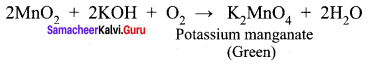2. Oxidation of potassium manganate to potassium permanganate:
Potassium manganate can be oxidised in two ways, either by chemical oxidation or electrolytic oxidation.

3. Chemical oxidation – In this method potassium manganate is treated with ozone (O3) or chlorine to get potassium permanganate.
$${ 2MnO }_{ 4 }^{ 2- }$$ + O3 + H2O → $${ 2MnO }_{ 4 }^{ 2- }$$ + 2OH + O2
$${ 2MnO }_{ 4 }^{ 2- }$$ + Cl2 → $${ 2MnO }_{ 4 }^{ 2- }$$ + 2Cl

4. Electrolytic oxidation: In this method aqueous solution of potassium manganate is electrolyzed in the presence of little alkali.
K2MnO4 $$\equiv$$ 2K+ + $${ 2MnO }_{ 4 }^{ 2- }$$
H2O $$\equiv$$ H+ + OH

Manganate ions are converted into permanganate ions at anode.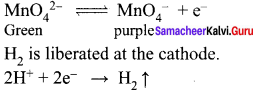The purple coloured solution is concentrated by evaporation and forms crystals of potassium permanganate on cooling.

Question 3.
Explain acidified KMnO4 is a powerful oxidising agent with 5 examples.
1. In the presence of dilute sulphuric acid, potassium permanganate acts as a very strong oxidising agent. Permanganate ions is converted into Mn2+ ion.
$${ MnO }_{ 4 }^{ – }$$ + 8H+ +5e → Mn2+ + 4H2O
Example:
1. Potassium permanganate oxidises ferrous salts to ferric salts.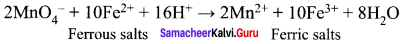2. Potassium permanganate oxidises iodide ions to iodine.
$${ 2MnO }_{ 4 }^{ – }$$ + 10I + 16H+ → 2Mn2+ + 5I2 + 8H2O

3. Potassium permanganate oxidises sulphide ion to sulphur.
$${ 2MnO }_{ 4 }^{ – }$$ + 5S2- + 16H+ → 2Mn2+ + 5S + 8H2O

4. Potassium permanganate oxidises oxalic acid to CO2.
$${ 2MnO }_{ 4 }^{ – }$$ + 5(COO)2- + 16H+ → 2Mn2+ + 10CO2 + 8H2O

5. Potassium permanganate oxidises alcohols to aldehyde.
2KMnO4 + 3H2SO4 + 5CH3CH2OH → 2K2SO4 + 2MnSO4 + 5CH3CHO + 8H2O

Question 4.
Explain about the oxidation state of Lanthanoids.

1. The common oxidation state of lanthanoids is +3. In addition to that some of the lanthanoids also show either +2 or +4 oxidation states.
2. Gd3+ and Lu3+ ions have extra stability, it is due to half filled and completely filled f-orbitals.
3. Cerium and terbium attain 4f7 and 4f14 configurations respectively in the +4 oxidation states.
4. Eu2+ and Yb2+ ions have exactly half filled and completely filled f orbitals.
5. Lu shows only +3 oxidation state.
6. Ce, Pr, Nd, Tb and Dy exhibit +3 and +4 oxidation states.
7. Nd, Sm, Eu, Tm, Yb exhibit +2 oxidation states also.

Common Errors:
Atomic number of d-block elements may get confused.

Rectifications:
Atomic number of d-block elements in group wise jumped as 18, 18, 18, 32.
e.g. Sc (Z = 21)
Y (Z = 39)
La (Z = 57)
Ac (Z = 89)

We hope the Class 12th Samacheer Kalvi Chemistry Solutions Chapter 4 Transition and Inner Transition Elements Book Solutions Answers Guide Solutions provided here help the students to get the best knowledge. Also, students can use our Samacheer Kalvi Class 12th Chemistry Solutions Chapter 4 Transition and Inner Transition Elements Questions and Answers for better practice. You can contact us at any moment by giving comments in the comment section. Keep checking updates by bookmarking our website.